# How to add algebraic fractions

Want to find out how to add algebraic fractions? We've provided a step-by-step guide of how to add algebraic fractions to teach you everything you need to know.## Step 1: Given two or more algebraic functions, to find their, make sure that they first share the same denominator

Hence, determine the least common denominator (LCD) shared by the algebraic fractions. This means that if we want to find the sum of 3a/(2a-1) and a/(4a-2), we’ll need to determine the LCD of 2a-1 and 4a-2.

Note that if the denominators are originally the same, then move on to Step 3 right away.

## Step 2: Rewrite algebraic fractions

Rewrite the algebraic fractions so that they have the LCD as their denominators

## Step 3: Cancel common factors

Cancel out common factors to find the simplified sum of the algebraic fractions

## Examples on adding algebraic fractions

Q1) Find the sum of the algebraic fractions 2a/(a+3) and 6/(a+3)

Since the denominators are the same, add the numerators directly.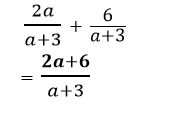Cancel out common factors to simplify the sum of the algebraic fraction.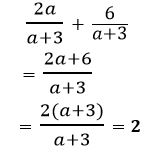Q2) What is the result if we add 3/(y+1) and 1/(5y+5) ? Since the denominators are not the same, determine the least common denominator (LCD) first before finding the sum.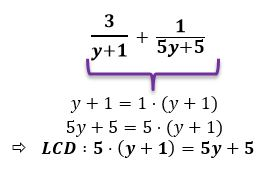Express the fractions so that they have 5y+5 as the denominator of the fractions.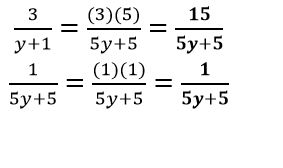Now that they have the same denominator, we can add the numerators directly.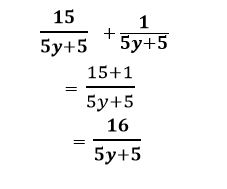#### Join today

The fastest way to practice

Unlock our complete testing platform and improve faster that ever.